output.to from Sideway
Algebra

Draft for Information Only

# Content

```  Quadratic Equations  Roots of Quadratic Equations  Quadratic Formula of Quadratic Equations  Discriminant of Quadratic Equations  Alternate Form of Quadratic Formula  Forms of Quadratic Function  Sign of Quadratic Expression```

## Quadratic Equations

A quadratic function is a polynomial function in a single variable, x, of the form equivalent to f(x)=ax2+bx+c with a≠0. The expression of a  quadratic function is therefore called a polynimial of degree 2, or the second order or second degree polynomial with the highest exponent of the single variable, x equal to 2. And a quadratic equation is any equation of the form equivalent to ax2+bx+c=0 by setting the quadratic function equal to zero. Therefore a quadratic equation is only a typical example of a quadratic function.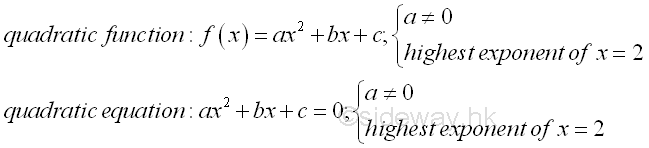## Roots of Quadratic Equations

The solutions of a quadratic equation is also called roots of a quadratic equation. Since a quadratic equation is a second order polynomial equation, a quadratic equation must has two solutions according to the fundamental theorem of algebra. In general, the solution may be both real, or both complex. Assume α and β are the roots of the quadratic equation, ax2+bx+c=0, the quadratic equation can then be written as (x-α)(x-β)=ax2-(α+β)x+αβ=0, imply (α+β)=-b/a and αβ=c/a by equating the coefficients of two polynomials.## Quadratic Formula of Quadratic Equations

Analytically, the solutions of quadratic equation can be found by the method of "completing the square". The idea of determining the roots of a quadratic equation is making use of the symmetry of the quadratic function g(x) about a vertical axis parallel to the y-axis by transforming the quadratic equation f(x)=0 into a symmetry quadratic function g(x). The obtained formula for calculating the roots of the quadratic equation is called the quadratic formula.   Imply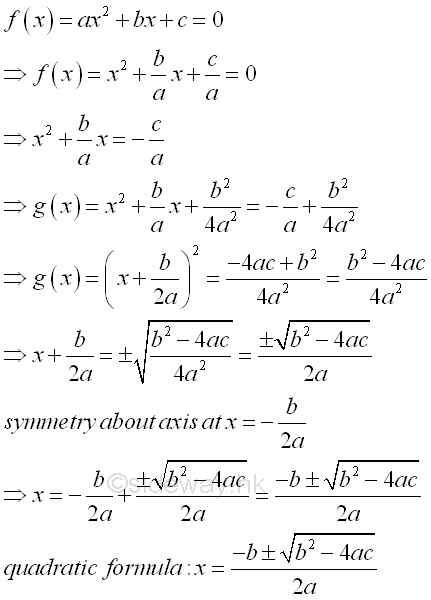Assuming α≥β then the values of the α and β are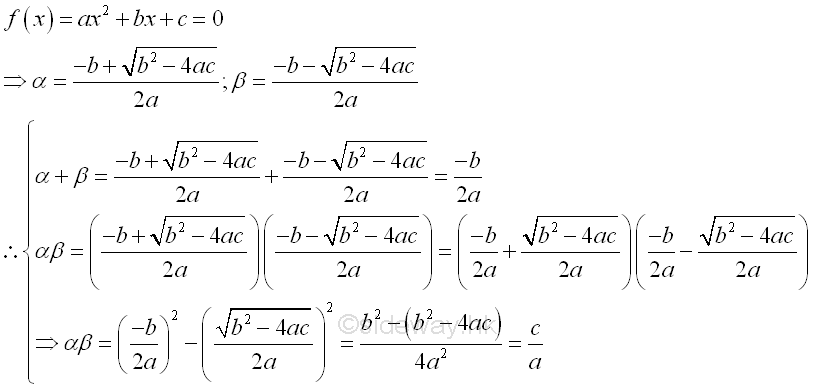## Discriminant of Quadratic Equations

According to the quadratic formula, the roots of a quadratic equation is charactered by the square root components of the quadratic formula. Depending on the value of expression (b2-4ac), the roots of a quadratic equation can be coincident, rational, real or complex numbers. And (b2-4ac) is therefore called the discriminant of the quadratic equation. In general, the discriminant, b2-4ac, can have four cases:

1. b2>4ac, imply discriminant, b2-4ac>0, if b2-4ac is a perfect or complete square then two distinct roots are rational.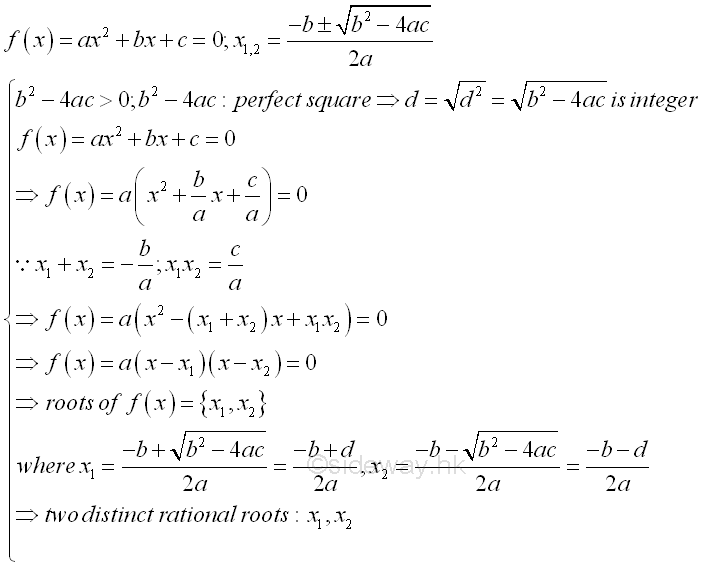2. b2>4ac, imply discriminant, b2-4ac>0, if b2-4ac is not a perfect or complete square then two distinct roots are real or irrational.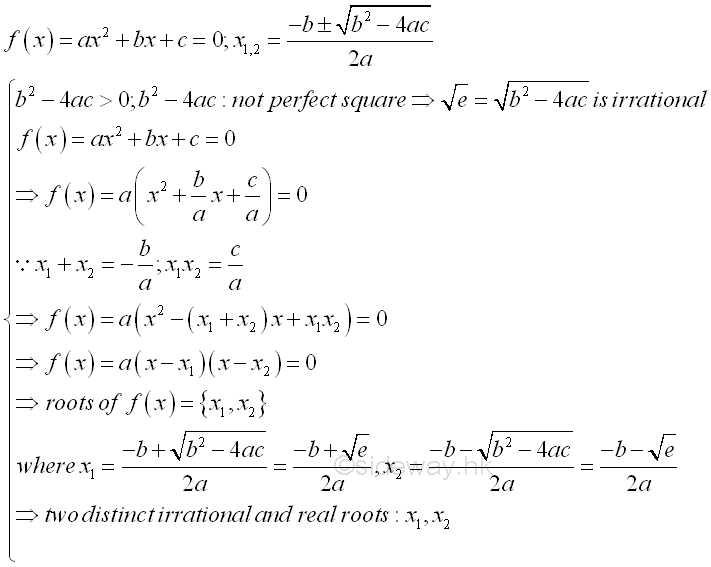3. b2=4ac, imply discriminant, b2-4ac=0, two roots are rational and coincident. That is two equal roots, one double root or one single solution.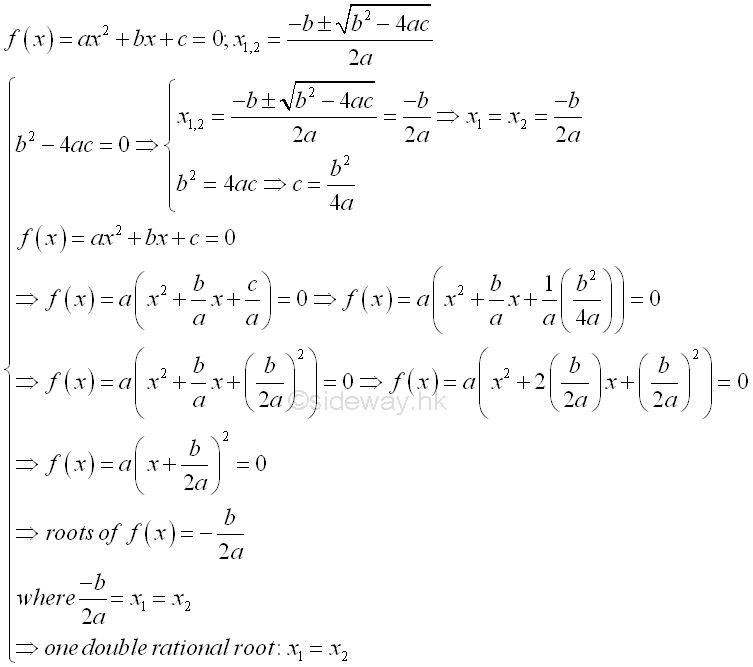4. b2<4ac, imply discriminant, b2-4ac<0, two distinct roots are complex or imaginary.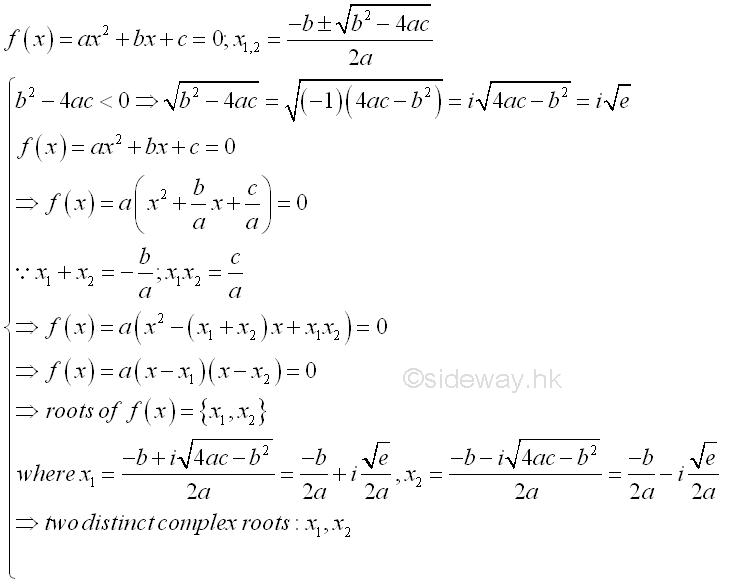## Alternate Form of Quadratic Formula

The quadratic equation is obtained by setting the quadratic function equal to zero and x is not equal to zero, the quadratic formula can be derived in an alternative way.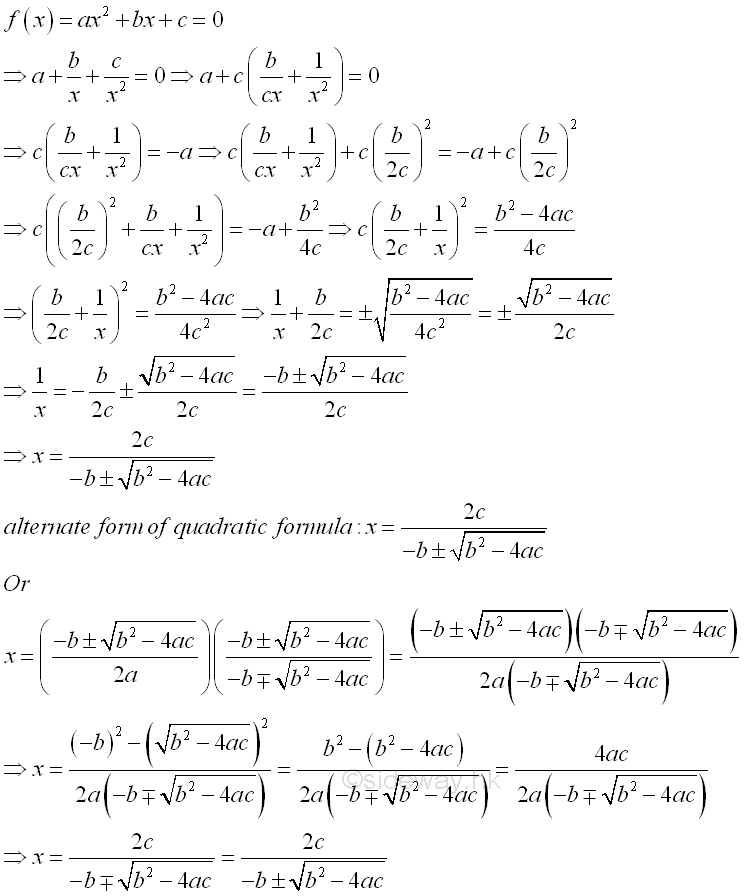## Forms of Quadratic Function

The quadratic function f(x)=ax2+bx+c is usually called the standard form of a quadratic function. Since a quadratic equation has two roots, the standard form of a quadratic function can be expressed in terms of the two roots,  f(x)=a(x-x1)(x-x2), called factored form.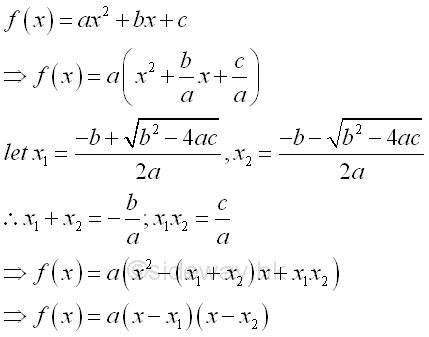Besides the quadratic function is symmetric about a line, the standard form of a quadratic function can be expressed in terms of the vertex of the function, f(x)=a(x-x0)2+y0, called vertex form.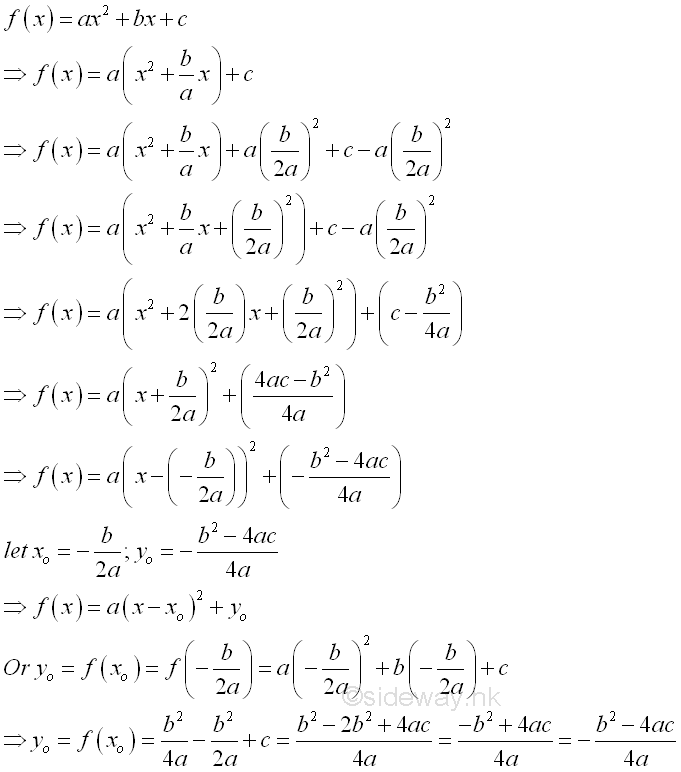## Sign of Quadratic Expression

Let E be the quadratic expression of the quadratic function f(x)=ax2+bx+c. If α and β are the roots of quadratic equation ax2+bx+c=0 and α≥β, then the sign of the quadratic expression can also be determined accordingly. In general, assuming the quadratic expression with two distinct real roots, when a is positive,  E is positive if x>α or x<β and E is negative if α>x>β. Or when a is negative, E is positive if α>x>β and E is negative if x>α or x<β. In other words, E has same sign as coefficient a for x>α or x<β, and E has opposite sign as coefficient a for α>x>β. This case can also be considered as the case b2-4ac>0 with two distinct real root for the corresponding quadratic equation. ImplyIn the case b2-4ac=0 with double real root  for the corresponding quadratic equation, this is a special condition of the general case where α equal to β and therefore the condition α>x>β becomes α=x=β is equal to zero. E has the same sign as the coefficient a.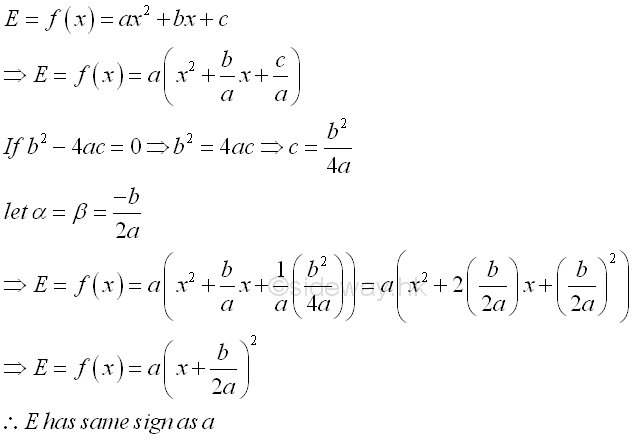In the case b2-4ac<0 with two distinct complex root  for the corresponding quadratic equation,  this is a special condition of the general case where quadratic expression, E, does not equal to zero for all real x. E has the same sign as the coefficient a..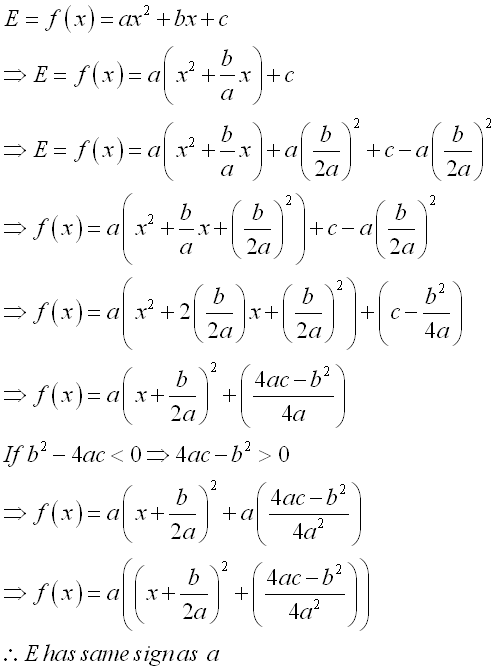©sidewayReferences

1. B. Joseph, 1978, University Mathematics: A Textbook for Students of Science & Engineering, Blackie & Son Limited, HongKong
2. Wheatstone, C., 1854, On the Formation of Powers from Arithmetical Progressions, Proceedings of The Royal Society of London, Vol 7, p145-151,, London
3. Stroud, K.A., 2001, Engineering Mathematics, Industrial Press, Inc, NY
4. Coolidge, J.L., 1949, The Story of The Binomial Theorem, The American Mathematical Monthly, Vol 56, No.3, Mar, pp147-157ID: 130500017 Last Updated: 2013/5/17 Revision: Ref:Home (5)

Business

Management

HBR (3)

Information

Recreation

Hobbies (7)

Culture

Chinese (1097)

English (336)

Reference (66)

Computer

Hardware (149)

Software

Application (187)

Digitization (24)

Numeric (19)

Programming

Web (648)CSS (SC)

ASP.NET (SC)

HTML

Knowledge Base

Common Color (SC)

Html 401 Special (SC)

OS (389)

MS Windows

Windows10 (SC)

.NET Framework (SC)

DeskTop (7)

Knowledge

Mathematics

Formulas (8)

Number Theory (206)

Algebra (20)

Trigonometry (18)

Geometry (18)

Calculus (67)

Complex Analysis (21)

Engineering

Tables (8)

Mechanical

Mechanics (1)

Rigid Bodies

Statics (92)

Dynamics (37)

Fluid (5)

Control

Acoustics (19)

Biology (1)

Geography (1)

Copyright © 2000-2019 Sideway . All rights reserved Disclaimers last modified on 10 Feb 2019